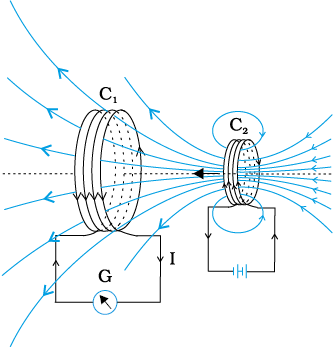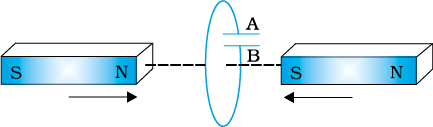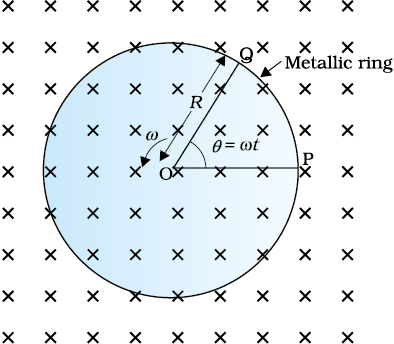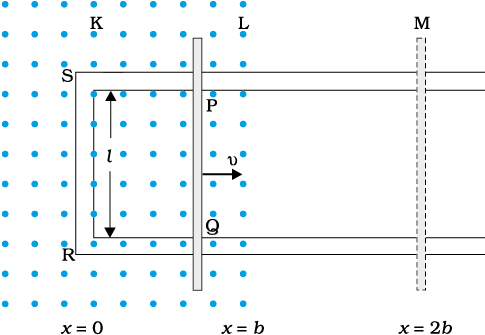Select Chapter Topics:A square loop of side 10 cm and resistance 0.5 $\Omega$ is placed vertically in the east-west plane. A uniform magnetic field of 0.10 T is set up across the plane in the north-east direction. The magnetic field is decreased to zero in 0.70 s at a steady rate. The magnitude of induced current during this time interval is:

1. 2 mA

2. 1 mA

3. 0.5 mA

4. 3 mASubtopic:  Faraday's Law & Lenz Law |
52%
To view explanation, please take trial in the course.
NEET 2023 - Target Batch - Aryan Raj Singh
Hints

A circular coil of radius 10 cm, 500 turns, and resistance 2 $\Omega$ is placed with its plane perpendicular to the horizontal component of the earth’s magnetic field. It is rotated about its vertical diameter through 180° in 0.25 s. The magnitude of the current induced in the coil is:

(The horizontal component of the earth’s magnetic field at the place is 3.0 × 10–5 T)

1. 3 mA

2. 2.5 mA

3. 5.2 mA

4. 1.9 mASubtopic:  Faraday's Law & Lenz Law |
55%
To view explanation, please take trial in the course.
NEET 2023 - Target Batch - Aryan Raj Singh
Hints

Consider the figure. What would you do to obtain a large deflection of the galvanometer?1. Use a rod made of soft iron inside the coil ${C}_{2}$

2. Connect the coil to a powerful battery

3. Move the arrangement rapidly towards the test coil ${C}_{1}$.

4. All of the aboveSubtopic:  Faraday's Law & Lenz Law |
83%
To view explanation, please take trial in the course.
NEET 2023 - Target Batch - Aryan Raj Singh
Hints

The figure shows planar loops of different shapes moving out of or into a region of a magnetic field which is directed normally to the plane of the loop away from the reader. Then:1 for the rectangular loop abcd, the induced current is clockwise. 2 for the triangular loop abc, the induced current is clockwise. 3 for the irregularly shaped loop abcd, the induced current is anti-clockwise. 4 none of these.Subtopic:  Faraday's Law & Lenz Law |
63%
To view explanation, please take trial in the course.
NEET 2023 - Target Batch - Aryan Raj Singh
Hints

Mark the correct statement:

 1 A closed-loop is held stationary in the magnetic field between the north and south poles of two permanent magnets held fixed. We can generate current in the loop by using very strong magnets. 2 A closed-loop moves normally to the constant electric field between the plates of a large capacitor. When it is wholly inside the region between the capacitor plates, a current is induced in the loop. 3 A closed-loop moves normally to the constant electric field between the plates of a large capacitor. When it is partially outside the plates of the capacitor, a current is induced in the loop. 4 None of these.Subtopic:  Faraday's Law & Lenz Law |
To view explanation, please take trial in the course.
NEET 2023 - Target Batch - Aryan Raj Singh
Hints

A rectangular loop and a circular loop are moving out of a uniform magnetic field region (as shown in the figure) to a field-free region with a constant velocity v. In which loop do you expect the induced emf to be constant during the passage out of the field region? The field is normal to the loops.1. Only in the case of the rectangular loop

2. Only in the case of the circular loop

3. In both cases

4. None of theseSubtopic:  Faraday's Law & Lenz Law |
61%
To view explanation, please take trial in the course.
NEET 2023 - Target Batch - Aryan Raj Singh
Hints

The polarity of the capacitor in the given figure is:1 The polarity of plate ‘B’ will be positive with respect to plate ‘A’. 2 The polarity of plate ‘A’ will be positive with respect to plate ‘B’. 3 The polarity of plate ‘A’ will be the same as that of plate ‘B’. 4 None of theseSubtopic:  Faraday's Law & Lenz Law |
63%
To view explanation, please take trial in the course.
NEET 2023 - Target Batch - Aryan Raj Singh
Hints

A metallic rod of 1 m length is rotated with a frequency of 50 rev/s, with one end hinged at the centre and the other end at the circumference of a circular metallic ring of radius 1 m, about an axis passing through the centre and perpendicular to the plane of the ring (as shown in the figure). A constant and uniform magnetic field of 1 T parallel to the axis is present everywhere. What is the emf between the centre and the metallic ring?1. 150 V

2. 130 V

3. 157 V

4. 133VSubtopic:  Motional emf |
70%
To view explanation, please take trial in the course.
NEET 2023 - Target Batch - Aryan Raj Singh
Hints

A wheel with $$10$$ metallic spokes each $$0.5$$ m long is rotated with a speed of $$120$$ rev/min in a plane normal to the horizontal component of earth’s magnetic field HE at a place. If $$H_E=0.4$$ G at the place, what is the induced emf between the axle and the rim of the wheel? ($$1$$ G=$$10^{-4}$$ T)
1. $$5.12\times10^{-5}$$ T
2. $$0$$
3. $$3.33\times10^{-5}$$
4. $$6.28\times10^{-5}$$Subtopic:  Motional emf |
64%
From NCERT
To view explanation, please take trial in the course.
NEET 2023 - Target Batch - Aryan Raj Singh
Hints

Refer to figure. The arm PQ of the rectangular conductor is moved from x = 0, outwards. The uniform magnetic field is perpendicular to the plane and extends from x = 0 to x = b and is zero for x > b. Only the arm PQ possesses substantial resistance r. Consider the situation when the arm PQ is pulled outwards from = 0 to x = 2b with a constant speed v. The induced emf is:Subtopic:  Motional emf |
50%
To view explanation, please take trial in the course.
NEET 2023 - Target Batch - Aryan Raj Singh
Hints